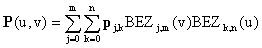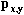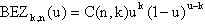## 7. Bezier surfaces

To create a Bťzier surface, We blend a mesh of Bťzier curves using the blending functionwhere j and k are points in parametric space andrepresents the location of the knots in real space. The Bťzier functions specify the weighting of a particular knot. They are the Bernstein coefficients. The definition of the Bťzier functions iswhere C(n,k) represents the binary coefficients. When u=0, the function is one for k=0 and zero for all other points. When we combine two orthogonal parameters, we find a Bťzier curve along each edge of the surface, as defined by the points along that edge. Bťzier surfaces are useful for interactive design and were first applied to car body design.

You can look at the Bezier surface from a 3x3 mesh, or the Bezier surface from a 4x4 mesh

### Further Information

Previous: Introduction to Surfaces
Next: B-Spline surfaces